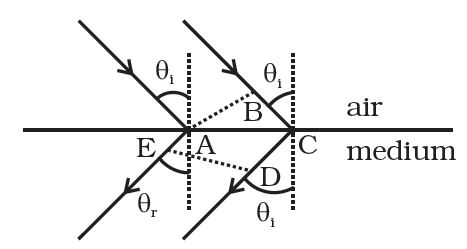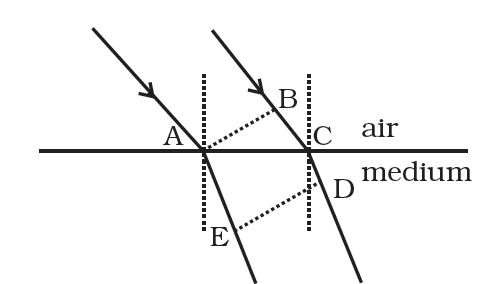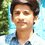# Metamaterials $\rightarrow n = - \sqrt{μ_r ε_r}$

The optical properties of a medium are governed by the relative permitivity $(ε_r)$ and relative permeability ($μ_r)$. The refractive index is defined as $\sqrt{μ_rε_r} = n$. For ordinary material $ε_r > 0$ and $μ_r > 0$ and the positive sign is taken for the square root.

In 1964, a Russian scientist V. Veselago postulated the existence of material with $ε_r < 0$ and $μ_r < 0$. Since then such ‘metamaterials’ have been produced in the laboratories and their optical properties studied.

For such materials $n = - \sqrt{μ_r ε_r}$ . As light enters a medium of such refractive index, the phases travel away from the direction of propagation.

(i) According to the description above show that if rays of light enter such a medium from air (refractive index =1) at an angle $θ$ in $2nd$ quadrant, then the refracted beam is in the $3rd$ quadrant.

(ii) Prove that Snell’s law holds for such a medium.

$(i)$ Suppose the postulate is true, then two parallel rays would proceed as shown in Figure. Assuming $ED$ shows a wave front then all points on this must have the same phase. All points with the same optical path length must have the same phase.Thus $- \sqrt{ε_rμ_r} AE = BC -\sqrt{ε_rμ_r}CD$ or $BC = \sqrt{ε_rμ_r}(CD - AE)$

$\rightarrow BC > 0, CD > AE$

As showing that the postulate is reasonable. If however, the light proceeded in the sense it does for ordinary material (viz. in the fourth quadrant, Fig. 2)Then $- \sqrt{ε_rμ_r} AE = BC -\sqrt{ε_rμ_r}CD$ or $BC = \sqrt{ε_rμ_r}(CD - AE)$

As $AE > CD, BC < O$, showing that this is not possible. Hence the postulate is correct.

$(ii)$ Prove yourself. $\ddot \smile$Note by Nishant Rai
5 years, 2 months ago

This discussion board is a place to discuss our Daily Challenges and the math and science related to those challenges. Explanations are more than just a solution — they should explain the steps and thinking strategies that you used to obtain the solution. Comments should further the discussion of math and science.

When posting on Brilliant:

• Use the emojis to react to an explanation, whether you're congratulating a job well done , or just really confused .
• Ask specific questions about the challenge or the steps in somebody's explanation. Well-posed questions can add a lot to the discussion, but posting "I don't understand!" doesn't help anyone.
• Try to contribute something new to the discussion, whether it is an extension, generalization or other idea related to the challenge.

MarkdownAppears as
*italics* or _italics_ italics
**bold** or __bold__ bold
- bulleted- list
• bulleted
• list
1. numbered2. list
1. numbered
2. list
Note: you must add a full line of space before and after lists for them to show up correctly
paragraph 1paragraph 2

paragraph 1

paragraph 2

[example link](https://brilliant.org)example link
> This is a quote
This is a quote
    # I indented these lines
# 4 spaces, and now they show
# up as a code block.

print "hello world"
# I indented these lines
# 4 spaces, and now they show
# up as a code block.

print "hello world"
MathAppears as
Remember to wrap math in $$ ... $$ or $ ... $ to ensure proper formatting.
2 \times 3 $2 \times 3$
2^{34} $2^{34}$
a_{i-1} $a_{i-1}$
\frac{2}{3} $\frac{2}{3}$
\sqrt{2} $\sqrt{2}$
\sum_{i=1}^3 $\sum_{i=1}^3$
\sin \theta $\sin \theta$
\boxed{123} $\boxed{123}$

Sort by:

Try these - Easy if you know about Metamaterials!

- 5 years, 2 months ago

a comprehension based on this was asked in $JEE$

- 5 years, 2 months ago